# Rounding Time Intervals to the Nearest Specified Time Increment in Microsoft Excel

In this article, we will learn how to round the time intervals to the nearest specified time increment. We will use “ROUND” function in Microsoft Excel to get the output.

ROUND: - This function is used for rounding a number to a specified number of digits.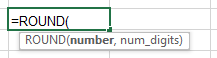For Example:Cell A1 is contains the number 456.25

To round the number, follow below given steps:-

• Write the formula in cell B1.
• =ROUND (A1, 0)
• Function will return 456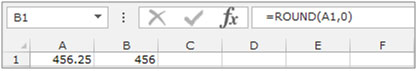Let’s take an example to understand how we can round interval time to the nearest specified time increment.

We have data in the range A1:B6 in which column A contains ‘Out Time’ and column B contains ‘In Time’. Now, we want to subtract each ‘Time In’ from its corresponding ‘Time Out’, and round the result to the nearest 30 minutes.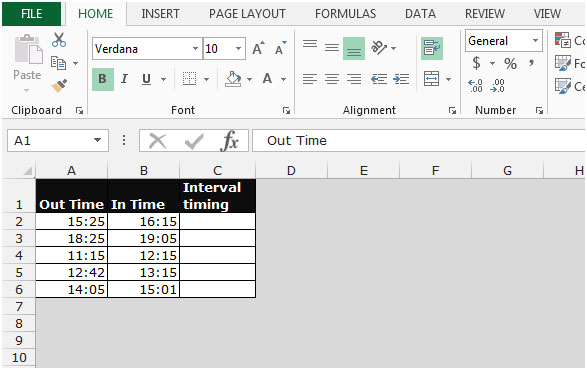• Write the formula in cell C2.
• =ROUND((B2-A2)/"0:30:00",0)*"0:30:00"
• Press Enter on your keyboard.
• The function will round the times down to the nearest 30 seconds.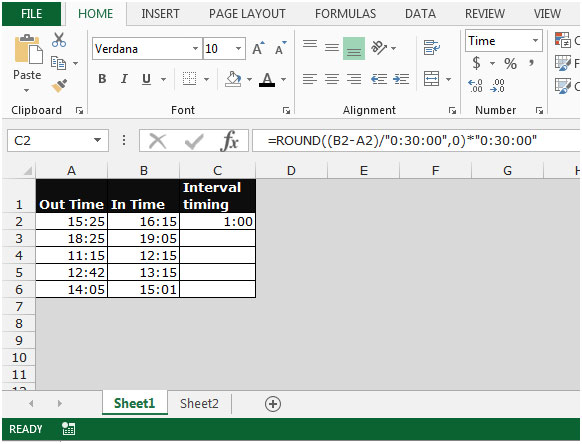• Copy the same formula by pressing the key Ctrl+C and paste in the range C3:C6 by pressing the key Ctrl+V on your keyboard.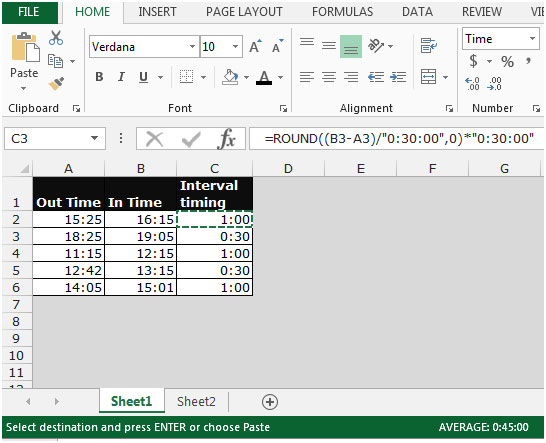This is the way to round time intervals to the nearest specified time increment in Microsoft Excel.